Latest Banking jobs   »

# Quantitative Aptitude Quiz For IBPS RRB PO/Clerk Mains 2022- 7th September

Directions (1-5): In each of these questions, two equations I and II are given. You have to solve both the equations and give answer.
(a) x>y
(b) x<y
(c) x≥y
(d) x≤y
(e) x=y or no relation can be established between x and y

Q1. I. 9y²+17y+8=0
II. x+y=4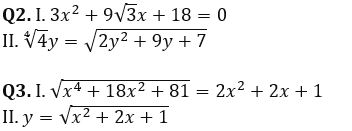Q4. I. 729x²-625x²=5096
II.√169 y³-10y³=2187

Q5. I. x²-y²=51
II. x+y=17

Directions (6-10): In the following questions two equations numbered (I) and (II) are given. You have to solve both equations and give answer accordingly.
(a) if x > y
(b) if x ≥ y
(c) if x < y
(d) if x ≤ y
(e) If x = y or the relationship cannot be established

Q6. I. 11x² – 74x + 120 = 0
II. 7y² – 70y + 168 = 0

Q7. I. 5x² + 14√6 x+ 54 = 0
II. 7y² – 94y – 165 = 0

Q8. I. 9x² – 45x + 44 = 0
II. 4y² – 39y + 90 = 0

Q9. I. 3x + 7y = 84
II. 7x + 3y = 76

Q10. I. x² – 16x – 105 = 0
II. 2y² + 25y + 77 = 0

Directions (11-15): In each of these questions, two equations (I) and (II) are given. You have to solve both the equations and give answer accordingly.
(a) if x>y
(b) if x≥y
(c) if x<y
(d) if x ≤y
(e) if x = y or no relation can be established between x and y.

Q11. I. 5x²-28x+39=0
II. 2y²-13y+20=0

Q12. I. 3x²-13x+14=0
II. 2y²-17y+33=0

Q13. I. 19x²-2x-17=0
II. y²-y-156=0

Q14. I. 7x²-22x+16=0
II. 46y²-35y-11=0

Q15. I. 15x²-11x-12=0
II. 20y²-49y+30=0

Solutions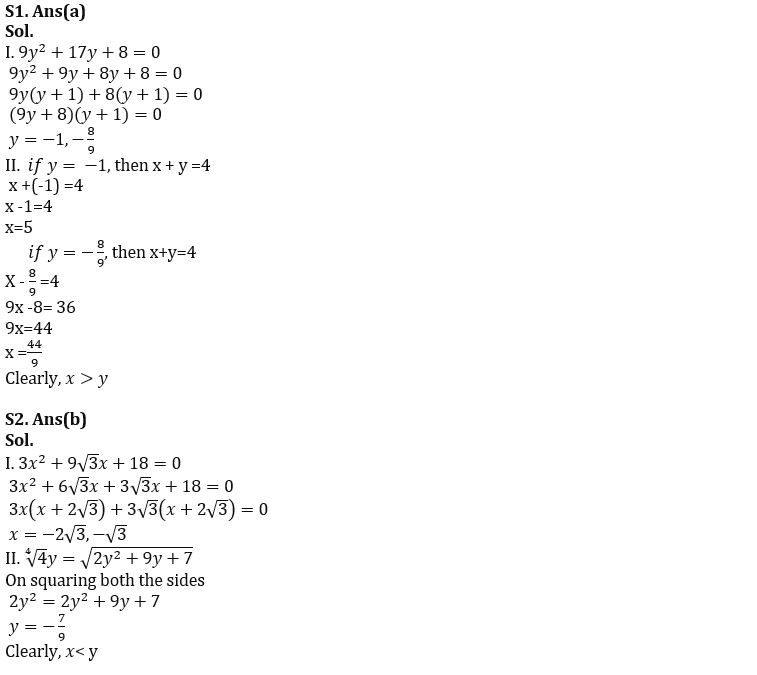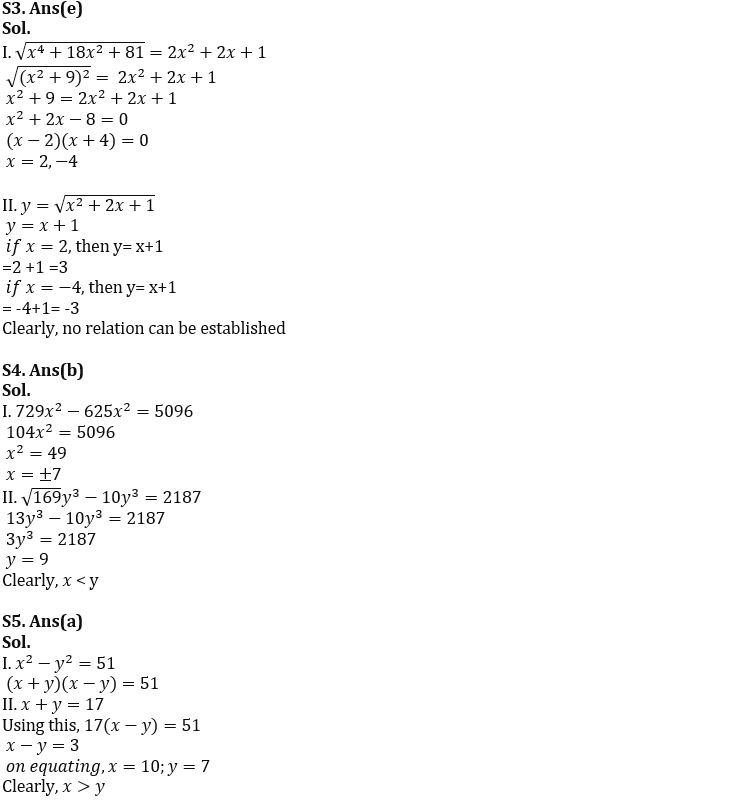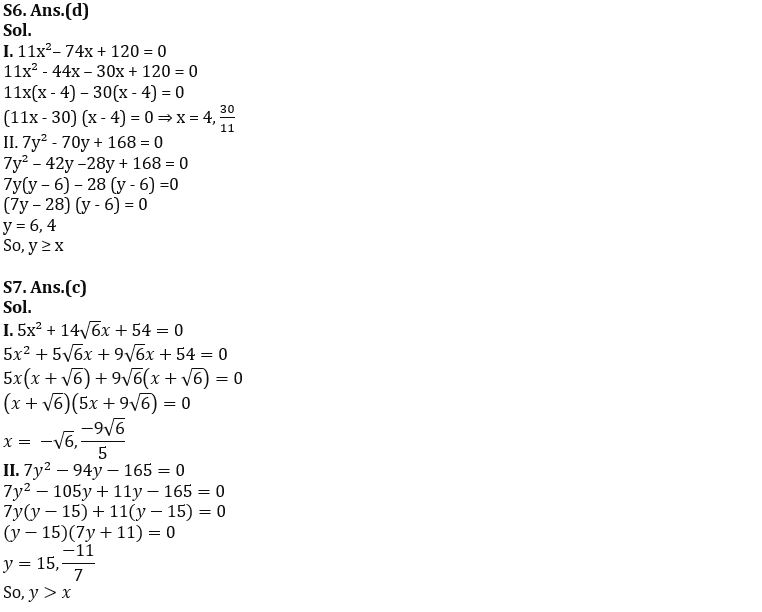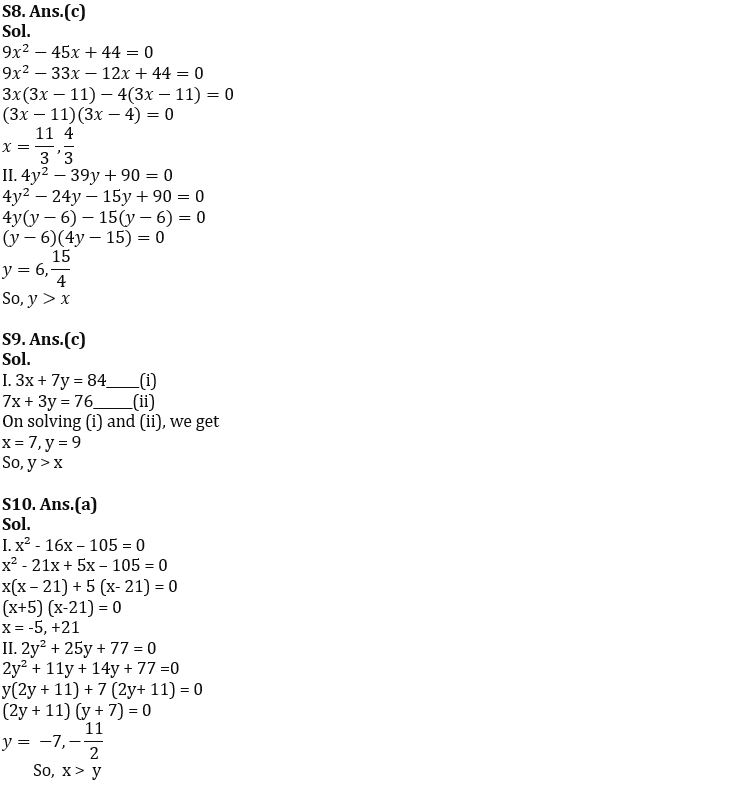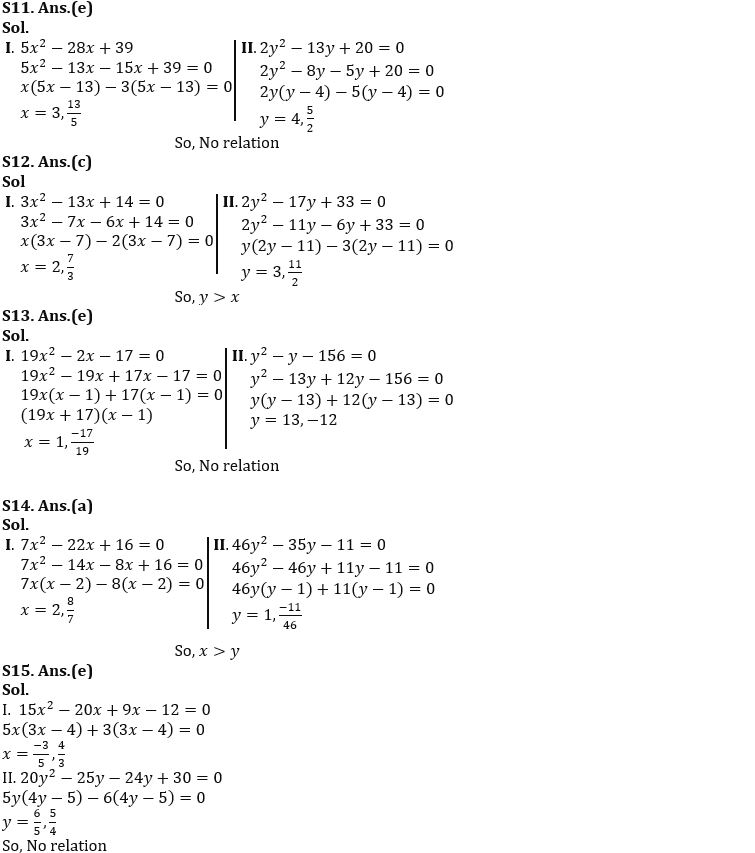#### Congratulations!Download Hindu Review of October 2021: Free PDF